Looking for VBA Tutorials?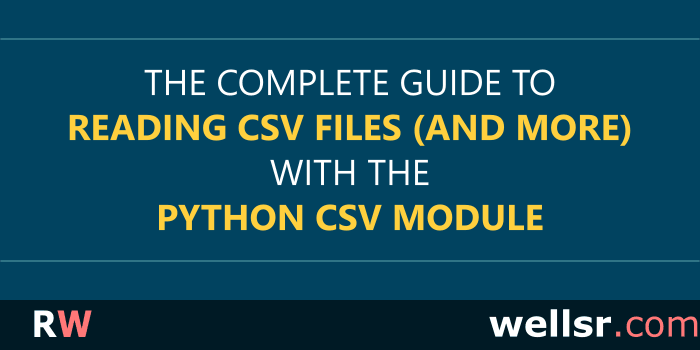#### Feb 22, 2019

Learn how to use Python to read CSV files with this tutorial describing the Python CSV reader function, the CSV DictReader class, and troubleshooting encoding errors.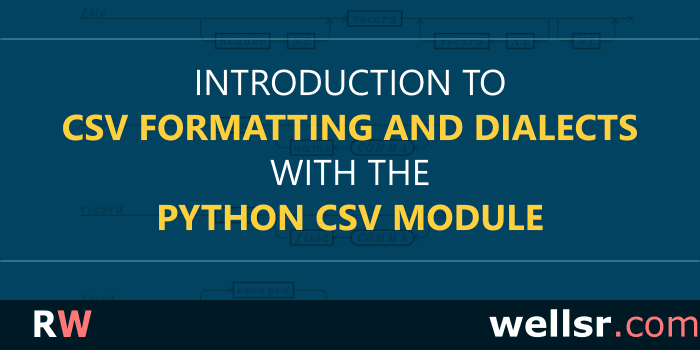#### Feb 15, 2019

The CSV data format lets you exchange data between applications in an implementation-independent fashion. This tutorial describes the syntax of the CSV dialects, and how you can use the Python CSV module to handle different CSV files.#### \$120.00 \$57.00

Want to learn more programming languages? We've combined each of our comprehensive VBA reference guides into a single bundle with over 200 tips and macros covering the 125 most important topics in VBA.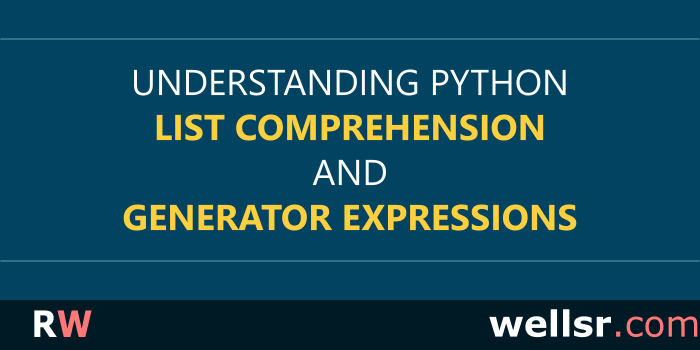#### Feb 1, 2019

Python comprehension expressions and generator expressions have a lot of similarities. This tutorial will discuss both comprehension and generator expressions in Python and explain how to use them.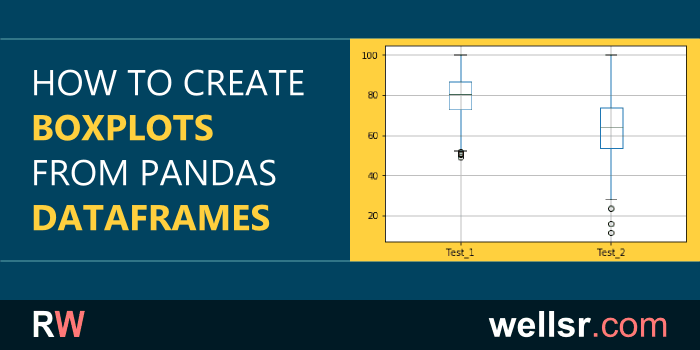#### Jan 19, 2019

This tutorial will teach you how to make a Pandas boxplot from a DataFrame. Boxplots, or box-and-whisker plots, help you approximate the distribution of your Pandas data.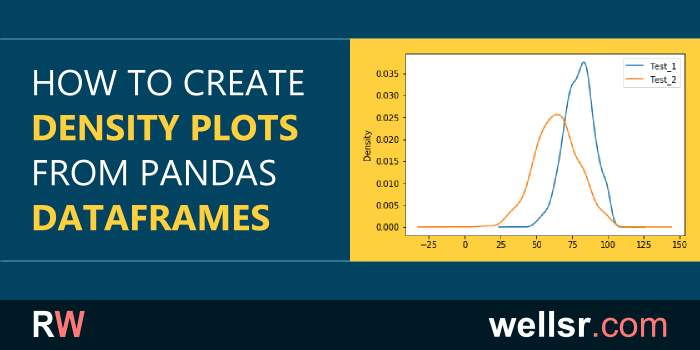#### Jan 11, 2019

This tutorial will teach you how to create density plots from Pandas DataFrames. Density plots, also called Kernel Density Estimation (KDE) plots are an important tool in data analysis.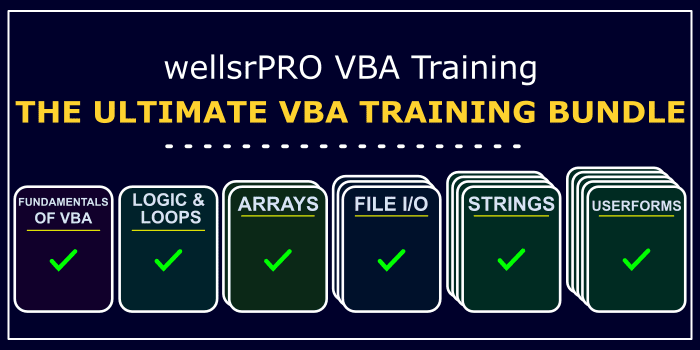#### \$120.00 \$57.00

We created a suite of 6 VBA cheat sheets with over 200 tips showing you everything you need to know to start making power Excel applications. Take a look!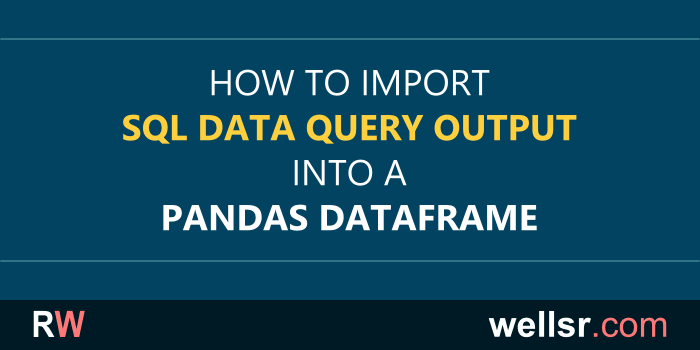#### Dec 17, 2018

Learn how to import SQL database queries into a Pandas DataFrame with this tutorial. To import a SQL query with Pandas, we'll first create a SQLAlchemy engine.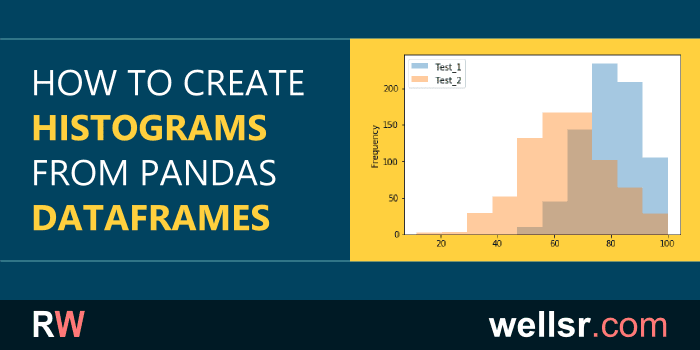#### Dec 10, 2018

This tutorial will teach you how to create histogram plots from Pandas DataFrames. Histograms are an important tool in data analysis and Python Pandas makes it easy.#### Nov 30, 2018

Learn how the Pandas Concat function is used to merge dataframes. The Pandas Concat function can merge dataframes by columns and rows. This Python tutorial contains examples of both.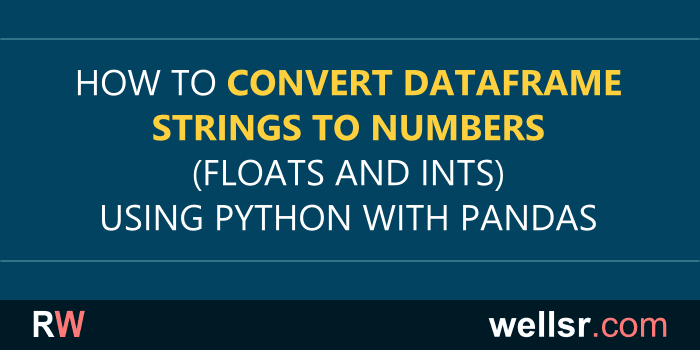#### Nov 9, 2018

This tutorial will show you how to convert Pandas DataFrame strings into floats or ints. Converting Pandas string data to numeric types is required before performing numeric calculations.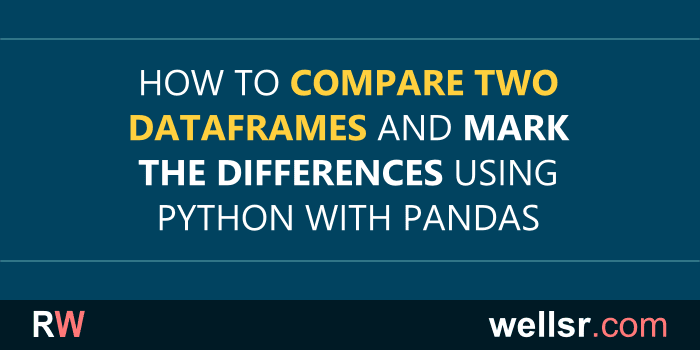#### Nov 6, 2018

This tutorial explains how to use Pandas to compare two DataFrames and identify their differences. Marking differences between DataFrames is valuable when analyzing data in Python.